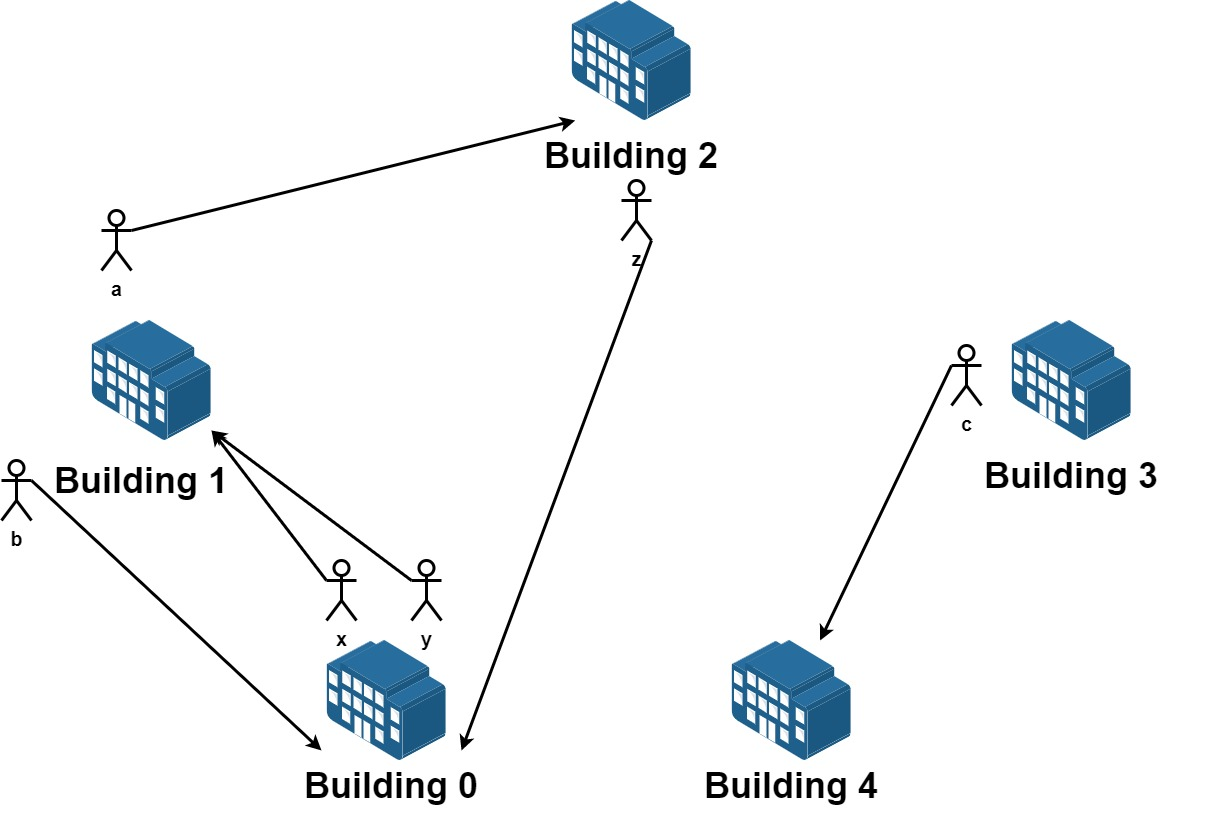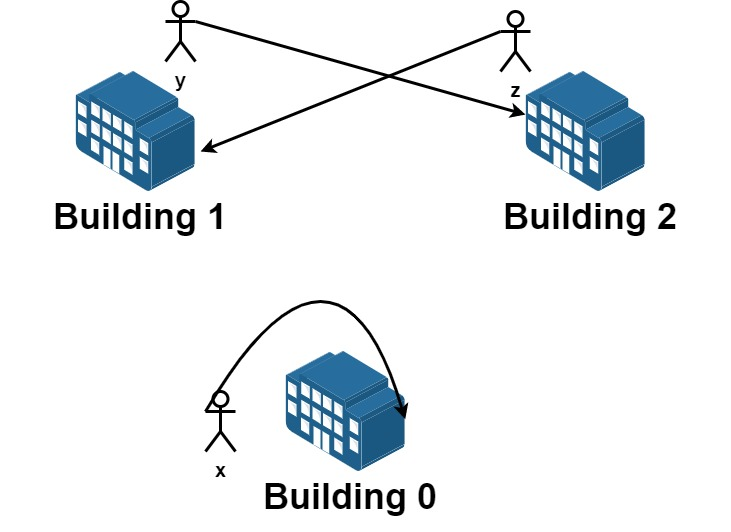##### Welcome to Subscribe On Youtube

Formatted question description: https://leetcode.ca/all/1601.html

# 1601. Maximum Number of Achievable Transfer Requests (Hard)

We have n buildings numbered from 0 to n - 1. Each building has a number of employees. It's transfer season, and some employees want to change the building they reside in.

You are given an array requests where requests[i] = [fromi, toi] represents an employee's request to transfer from building fromi to building toi.

All buildings are full, so a list of requests is achievable only if for each building, the net change in employee transfers is zero. This means the number of employees leaving is equal to the number of employees moving in. For example if n = 3 and two employees are leaving building 0, one is leaving building 1, and one is leaving building 2, there should be two employees moving to building 0, one employee moving to building 1, and one employee moving to building 2.

Return the maximum number of achievable requests.

Example 1:Input: n = 5, requests = [[0,1],[1,0],[0,1],[1,2],[2,0],[3,4]]
Output: 5
Explantion: Let's see the requests:
From building 0 we have employees x and y and both want to move to building 1.
From building 1 we have employees a and b and they want to move to buildings 2 and 0 respectively.
From building 2 we have employee z and they want to move to building 0.
From building 3 we have employee c and they want to move to building 4.
From building 4 we don't have any requests.
We can achieve the requests of users x and b by swapping their places.
We can achieve the requests of users y, a and z by swapping the places in the 3 buildings.


Example 2:Input: n = 3, requests = [[0,0],[1,2],[2,1]]
Output: 3
Explantion: Let's see the requests:
From building 0 we have employee x and they want to stay in the same building 0.
From building 1 we have employee y and they want to move to building 2.
From building 2 we have employee z and they want to move to building 1.
We can achieve all the requests. 

Example 3:

Input: n = 4, requests = [[0,3],[3,1],[1,2],[2,0]]
Output: 4


Constraints:

• 1 <= n <= 20
• 1 <= requests.length <= 16
• requests[i].length == 2
• 0 <= fromi, toi < n

Related Topics:
Dynamic Programming

## Solution 1.

Check all the different combinations of the requests.

For each combination, check if the sum of indegree is the same as the sum of outdegree. If they are equal, it’s a valid combination.

Return the maximum size of the valid combinations.

// OJ: https://leetcode.com/problems/maximum-number-of-achievable-transfer-requests/
// Time: O(2^M * (M + N))
// Space: O(N)
class Solution {
public:
int maximumRequests(int n, vector<vector<int>>& requests) {
int m = requests.size(), ans = 0, g = {};
memset(g, 0, sizeof(g));
int cnt = 0;
for (int i = 0; i < m; ++i) {
if (mask & (1 << i)) {
cnt++;
auto &r = requests[i];
int u = r, v = r;
g[u]++;
g[v]--;
}
}
bool good = true;
for (int i = 0; i < n && good; ++i) good = g[i] == 0;
if (good) ans = max(ans, cnt);
}
return ans;
}
};


## Solution 2. DFS

// OJ: https://leetcode.com/problems/maximum-number-of-achievable-transfer-requests/
// Time: O(2^M * N)
// Space: O(N)
class Solution {
int ans = 0;
vector<int> g;
void dfs(vector<vector<int>> &R, int i, int cnt) {
if (i == R.size()) {
for (int n : g) {
if (n) return;
}
ans = max(ans, cnt);
return;
}
int u = R[i], v = R[i];
g[u]++;
g[v]--;
dfs(R, i + 1, cnt + 1);
g[u]--;
g[v]++;
dfs(R, i + 1, cnt);
}
public:
int maximumRequests(int n, vector<vector<int>>& R) {
g.assign(n, 0);
dfs(R, 0, 0);
return ans;
}
};

• class Solution {
public int maximumRequests(int n, int[][] requests) {
int maximumRequestsCount = 0;
int requestsLength = requests.length;
int counts = 1 << requestsLength;
for (int i = 0; i < counts; i++) {
boolean[] selections = getSelections(requestsLength, i);
if (isPossible(n, requests, selections)) {
int requestsCount = 0;
for (boolean selection : selections) {
if (selection)
requestsCount++;
}
maximumRequestsCount = Math.max(maximumRequestsCount, requestsCount);
}
}
return maximumRequestsCount;
}

public boolean[] getSelections(int requestsLength, int index) {
boolean[] selections = new boolean[requestsLength];
for (int i = 0; i < requestsLength && index > 0; i++) {
selections[i] = index % 2 == 1;
index /= 2;
}
return selections;
}

public boolean isPossible(int n, int[][] requests, boolean[] selections) {
int[] outdegrees = new int[n];
int[] indegrees = new int[n];
int requestsLength = requests.length;
for (int i = 0; i < requestsLength; i++) {
if (selections[i]) {
int[] request = requests[i];
int from = request, to = request;
outdegrees[from]++;
indegrees[to]++;
}
}
for (int i = 0; i < n; i++) {
if (outdegrees[i] != indegrees[i])
return false;
}
return true;
}
}

############

class Solution {
public int maximumRequests(int n, int[][] requests) {
int ans = 0;
if (ans < cnt && check(mask, requests)) {
ans = cnt;
}
}
return ans;
}

private boolean check(int x, int[][] requests) {
int[] d = new int;
for (int i = 0; i < requests.length; ++i) {
if (((x >> i) & 1) == 1) {
int f = requests[i];
int t = requests[i];
--d[f];
++d[t];
}
}
for (int v : d) {
if (v != 0) {
return false;
}
}
return true;
}
}

• // OJ: https://leetcode.com/problems/maximum-number-of-achievable-transfer-requests/
// Time: O(2^M * (M + N))
// Space: O(N)
class Solution {
public:
int maximumRequests(int n, vector<vector<int>>& A) {
int M = A.size(), ans = 0, cnt = {};
auto valid = [&](int m) {
memset(cnt, 0, sizeof(cnt));
for (int i = 0; i < M; ++i) {
if ((m >> i & 1) == 0) continue;
--cnt[A[i]];
++cnt[A[i]];
}
for (int i = 0; i < n; ++i) {
if (cnt[i]) return false;
}
return true;
};
for (int m = 1; m < (1 << M); ++m) {
if (valid(m)) ans = max(ans, __builtin_popcount(m));
}
return ans;
}
};

• class Solution:
def maximumRequests(self, n: int, requests: List[List[int]]) -> int:
def check(x):
d =  * n
for i, (f, t) in enumerate(requests):
if (x >> i) & 1:
d[f] -= 1
d[t] += 1
return all(v == 0 for v in d)

ans, m = 0, len(requests)
for mask in range(1 << m):
if cnt > ans and check(mask):
ans = cnt
return ans


• func maximumRequests(n int, requests [][]int) int {
check := func(x int) bool {
d := make([]int, n)
for i, r := range requests {
if (x>>i)&1 == 1 {
d[r]--
d[r]++
}
}
for _, v := range d {
if v != 0 {
return false
}
}
return true
}

ans, m := 0, len(requests)
if ans < cnt && check(mask) {
ans = cnt
}
}
return ans
}

• /**
* @param {number} n
* @param {number[][]} requests
* @return {number}
*/
var maximumRequests = function (n, requests) {
function check(x) {
let d = new Array(n).fill(0);
for (let i = 0; i < m; ++i) {
if ((x >> i) & 1) {
const [f, t] = requests[i];
d[f]--;
d[t]++;
}
}
for (const v of d) {
if (v) {
return false;
}
}
return true;
}
let ans = 0;
let m = requests.length;
if (ans < cnt && check(mask)) {
ans = cnt;
}
}
return ans;
};


• function maximumRequests(n: number, requests: number[][]): number {
const m = requests.length;
let ans = 0;
const check = (mask: number): boolean => {
const cnt = new Array(n).fill(0);
for (let i = 0; i < m; ++i) {
if ((mask >> i) & 1) {
const [f, t] = requests[i];
--cnt[f];
++cnt[t];
}
}
return cnt.every(v => v === 0);
};
if (ans < cnt && check(mask)) {
ans = cnt;
}
}
return ans;
}

function bitCount(i: number): number {
i = i - ((i >>> 1) & 0x55555555);
i = (i & 0x33333333) + ((i >>> 2) & 0x33333333);
i = (i + (i >>> 4)) & 0x0f0f0f0f;
i = i + (i >>> 8);
i = i + (i >>> 16);
return i & 0x3f;
}